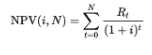# Financial Summary PMI Metrics

Note: Must be using Financial Summary for these fields to be meaningful. Must be member of Internal Cost Visibility team to view these fields.

Planview provides a set of out-of-the-box fields to use in conjunction with the financial summary to provide standard metrics based on a project's financials. The fields can be displayed on a project's Details and Additional Details sections, and can be included in reports.

The fields consider values from the project's financial summary as well as the project's % Complete method when performing calculations.

Note that the metrics take into account cost only - revenue is excluded. As a result all calculations use positive values, although the results can be negative.

The following is the list of fields and their definitions. ALL of these calculations are based on the Financial Summary structure rollup/representation.

PMI Metric Field Definition
Actual Cost (AC) Calculated as total Actuals up to the current date
Budget at Completion (BAC) The total project budget
Cost Performance Index (CPI) Earned Value divided by Actual Cost. A value less than 1 indicates that the project is over budget.
Cost Variance (CV) Earned Value – Actual Cost
Earned Value (EV) The Budget at Completion multiplied by the % complete of the project
Estimate at Completion (EAC) Calculated based on selected settings. See Setting Up ETC/EAC/NPV Calculations.
Estimate to Complete (ETC) Calculated based on selected settings. See Setting Up ETC/EAC/NPV Calculations.
Net Present Value (NPV)

The difference between the present value of cash inflows and the present value of cash outflows over a period of time given a discount rate:The first period (= 0) is the initial investment. Projects can have up to three NPV fields.

NOTE

Cash flow is never prorated based on the Cash flow start date. The calculation always considers the entire month, quarter, or year that the start date falls into. For example, if you specify a Cash flow start date of May 2, 2022, and Calculate for:

• 12 Months - the calculation will include May 2022 through April 2023, the initial investment period is May 2022
• 3 Quarters - the calculation will include Q2 (the quarter May 2022 falls into) starting with April 2022 through Q4 ending with December 2022, the initial investment period is Q2
• 4 Years - the calculation will include 2022 (the year May 2022 falls into) starting with January 2022 through December 2025, the initial investment period is 2022

Planned Value (PV) The total budgeted amount through the current date
Return On Investment (ROI) (Forecasted Total Revenue – Forecasted Total Cost) / Forecasted Total Cost, expressed as a %
Schedule Performance Index (SPI) Earned Value divided by Planned Value. A value less than 1 indicates that the project is running behind schedule.
Schedule Variance (SV) Earned Value – Planned Value
To Complete Performance Index (TCPI) (Budget at Completion – Earned Value) / (Budget at Completion – Actual Cost)
Variance at Completion (VAC) Budget at Completion – Estimate at Completion

### Project and Portfolio Roll Up Fields

The following fields are standard roll up fields for Projects and Portfolios. They roll up data from the Total Budget, Total Actuals, and Total Forecast financial summary fields. If you need to create a roll up for a different financial summary field, you can follow the guide in Working with the Financial Summary.

#### Project Roll Up Fields

 Field Name Description Total Budget (Financial Summary) The sum of all budget values in the project financial summary. Total Forecast (Financial Summary) The sum of all forecast values in the project financial summary. Total Actuals (Financial Summary) The sum of all actuals values in the project financial summary.

#### Portfolio Roll Up Fields

 Field Name Description Total Project Budget (Financial Summary) The sum of all budget values in the associated project financial summaries. Total Project Forecast (Financial Summary) The sum of all forecast values in the associated project financial summaries. Total Project Actuals (Financial Summary) The sum of all actuals values in the associated project financial summaries.